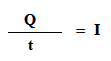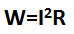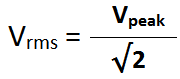## Electrical Power Theory and Formula

Electrical energy is the most usable energy in the modern day. Nowadays the whole world is dependent on electrical energy. Electrical power can be transferred easily and also can be distributed easily. In modern days we cannot live without electrical energy. We use electrical power or electrical energy from the start of the day to the end of the day for lighting, heating, and many other works. The conversion of electrical energy into any other energy is very easy. Today we are going to know all the Electrical Power Theory and Formula.

### What is Electrical Power?

#### Electric Power Definition:

Electrical power is the rate of transfer of electrical energy into other energy per unit of time. In other words, it can be called that the electrical power is the rate of electrical energy consumed by load per unit time.

The standard unit of electrical power is Watt.

According to the definition of electrical power, we can say the basic electrical power formula isHere, W= power in watt
Q= charge in coulombs
V= voltage or electrical pressure in volt
t=time is sec

We know that the rate of flow of charge per unit time is called current(I)This is the basic formula of electrical power.
According to the OHM's law, we know that V= I*R
So we can represent the electrical power formula by current(I) and Resistance(R) that is,We can also represent the formula by voltage(V) and Resistance(R) that is,
W=V2R

There are two types of electrical power available according to the nature of the Current,
1. Direct Current (DC)
2. Alternating Current(AC)
The DC Power and AC Power have different formulas.

### DC Power Formula

DC has a constant value which means the magnitude and direction of the current do not change with time.
Actually, the electrical power depends upon three important things,
1. Voltage
2. Current
3. The angle between voltage and current
The angle between the voltage and current exists when the circuit consists of an inductor or capacitor. If an electrical circuit consists the only a resistor then naturally there will be no angle between the voltage and current but if the circuit consists inductor or capacitor there may be some angle between the voltage and current.
In the DC circuit, there is no angle between voltage and current even if the circuit consists of Inductors or capacitors because the inductance and capacitance effect does not exist in the DC supply.
So the DC power will be the product of the voltage and current.
So the DC power formula is, P = V*I

Here, P= electrical power in watts
V= voltage in volts
I= electric current in amps.

Power Factor: Power factor is a very important thing when we talk about electric power. Power Factor is the cosine of the angle between voltage and current in an electrical circuit. It is defined as cos φ or cosθ. Here φ or θ is the angle between voltage and current.

#### What is the Power Factor of DC?

As we know the angle(φ) between voltage and current in a DC circuit is Zero(0). So the power factor of DC will be cos 0 = 1
As the power factor of the DC is 1 (one) it can not affect the power formula and we always say that the DC has no power factor.

Read Also: Impedance Formula and Theory Explanation

### Electrical Power Formula For AC

Now let's know the AC power formula. As the magnitude and direction of the current of AC change with time so the electrical power formula is different. If an AC circuit consists the only a resistor then naturally the angle between current and voltage will be Zero but if consists inductor or capacitor there may be some angle between voltage and current.

There are three types of AC Power,
1. Apparent Power
2. Active Power
3. Reactive Power

#### Apparent Power:

The simple product of the voltage and current of an AC circuit is called Apparent Power. But remember that the value of the voltage and current should be the RMS value.
The Apparent power is denoted by 'S'
So the formula of apparent power,
S=Vrms * Irms

The RMS value of the voltage can be found in the equation,The RMS value of the current can be found in the equation,If the AC circuit only consists resistor then the power can be derived by this equation or in other words for a pure resistive circuit the apparent is equal to the active power.
The unit of Apparent power is Volt-Ampere.

#### Active Power:

It is the power which actually consumed by the AC Load consisting of resistance, inductance, and capacitance. It is denoted by 'P'
Active Power takes the cosine of the angle between the voltage and current into account.
So the active power formula is,
P=Vrms * Irms * cos φ

φ is the angle between voltage and current
The unit of Active power is Watt

#### Reactive Power:

The power dissipated by the circuit element reactance is called Reactive Power. Suppose an electrical AC circuit consists the only a resistor then we can say that the circuit does not have any reactance but if the circuit consists of inductance or reactance then the circuit will have reactance.
The reactive power is denoted by 'Q'
The formula of Reactive power is,
Q=Vrms * Irms * Sin φ

The relation between Apparent power, active power, and reactive power can be written as below,#### Electrical Power Triangle:

The electrical power triangle consists of,
1.  Active power in 'X' axis
2. Reactive power in 'Y' axis
3. Apparent power in 'Z' axis

Thank you for visiting the website. keep visiting for more updates.
Electrical Power Theory and Formula ExplanationReviewed by Author on January 01, 2019 Rating: 5